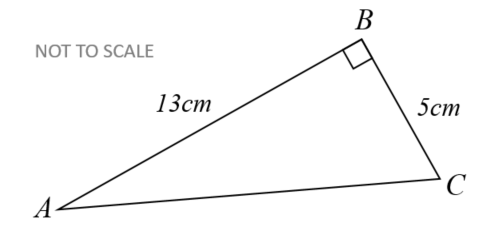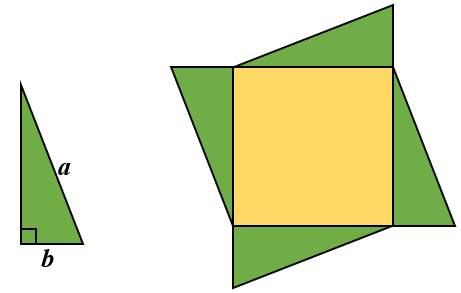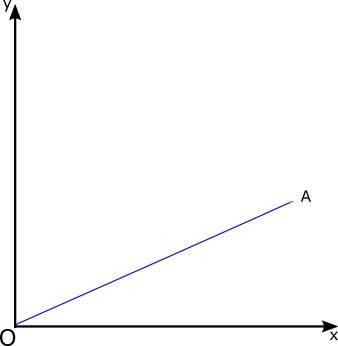# Exam-Style Questions on Pythagoras

## Problems on Pythagoras adapted from questions set in previous Mathematics exams.

### 1.

GCSE Higher

$$ABC$$ is a right-angled triangle.

John has a method for finding the length of $$AC$$$$AC^2 = AB^2 - BC^2$$ $$AC^2 = 13^2 - 5^2$$ $$AC^2 = 169 - 25$$ $$AC^2 = 144$$ $$AC = \sqrt{144}$$ $$AC = 12$$

John's answer of $$12cm$$ is not correct.

(a) What mistake has he made with his method?

(b) Show the correct method and the correct answer to 3 significant figures.

### 2.

GCSE Higher

An isosceles triangle shaped frame is made from four pieces of metal. The frame has a height of 8 metres and a base of length 12 metres.

The weight of the metal is 2.5kg per metre. Calculate the total weight of the metal in the frame.### 3.

GCSE Higher

Four copies of a green right-angled triangle are used to enclose a yellow square.Find the area of the yellow square if the longest side of the green triangle is of length $$a$$ cm and the shortest side is $$b$$ cm.

### 4.

GCSE Higher

The gradient of the line joining the origin to the point A is $$\frac12$$

The distance between A and the origin is $$\sqrt{2205}$$

What are the coordinates of A?If you would like space on the right of the question to write out the solution try this Thinning Feature. It will collapse the text into the left half of your screen but large diagrams will remain unchanged.

The exam-style questions appearing on this site are based on those set in previous examinations (or sample assessment papers for future examinations) by the major examination boards. The wording, diagrams and figures used in these questions have been changed from the originals so that students can have fresh, relevant problem solving practice even if they have previously worked through the related exam paper.

The solutions to the questions on this website are only available to those who have a Transum Subscription.

Exam-Style Questions Main Page

Search for exam-style questions containing a particular word or phrase:

To search the entire Transum website use the search box in the grey area below.